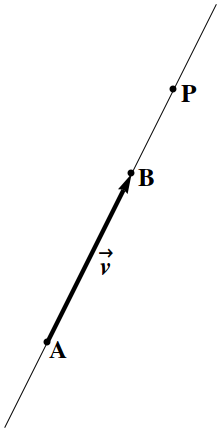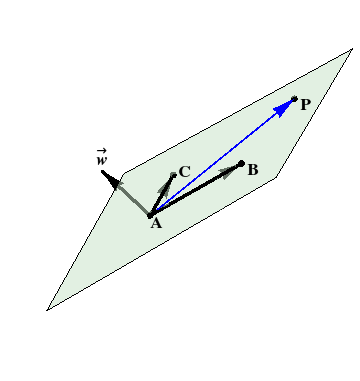## Section1.20Lines and Planes

Two points $$A$$ and $$B$$ determine a line. But there are also other ways to describe a line. Rather than specifying two points, we can specify just one ($$A$$), then give a vector $$\vv$$ along the line.Figure 1.20.1. The geometry behind the vector description of a line.

So let $$\AA$$ be the vector from the origin to the point $$A\text{,}$$ and $$\vv$$ be a vector from $$A$$ that points along the line. Then any other point $$P$$ on the line can be reached by going to $$A$$ along $$\AA\text{,}$$ then going along $$\vv\text{.}$$ Thus, the vector

\begin{equation} \rr(u) = \AA + \vv\,u\tag{1.20.1} \end{equation}

from the origin to $$P$$ provides a vector parameterization of the line. Equivalently, if

\begin{align*} \AA \amp = a_x\,\xhat + a_y\,\yhat + a_z\,\zhat ,\\ \vv \amp = v_x\,\xhat + v_y\,\yhat + v_z\,\zhat , \end{align*}

then the line is given by the parametric equations

\begin{align*} x \amp = a_x + v_x\,u ,\\ y \amp = a_y + v_y\,u ,\\ z \amp = a_z + v_z\,u , \end{align*}

which gives the coordinates $$(x,y,z)$$ of the point $$P$$ in terms of the parameter $$u\text{.}$$ A given line can have many parameterizations, depending not only on the choice of the point $$A$$ on the line and vector $$\vv$$ along the line, but also on the choice of parameter $$u\text{.}$$

How do two points determine a line? Using vector addition, you can think of $$\vv$$ as $$\BB-\AA\text{,}$$ and use the vector description above, as shown in Figure 1.20.1.

It takes three points $$A\text{,}$$ $$B\text{,}$$ $$C$$ to determine a plane, but again there are also other descriptions. The orientation of a line is given by a vector $$\vv$$ along the line. By contrast, the orientation of a plane is given by a vector $$\ww$$ perpendicular to the plane. A plane can therefore be specified by giving a point $$A$$ and a normal vector $$\ww$$ to the plane at the point $$A\text{.}$$Figure 1.20.2. The geometry behind the vector description of a plane.

If $$\rr$$ is the vector from the origin to $$P\text{,}$$ then $$\rr-\AA$$ is a vector in the plane, as shown in Figure 1.20.2. If $$\ww$$ is perpendicular to the plane, then it must be perpendicular to any vector in the plane. In particular, it must be perpendicular to $$\rr-\AA\text{,}$$ so that

\begin{equation} (\rr-\AA) \cdot \ww = 0\tag{1.20.2} \end{equation}

or equivalently

\begin{equation} \rr \cdot \ww = \AA\cdot\ww .\tag{1.20.3} \end{equation}

Inserting the components

\begin{align*} \rr \amp = x\,\xhat + y\,\yhat + z\,\zhat ,\\ \ww \amp = w_x\,\xhat + w_y\,\yhat + w_z\,\zhat , \end{align*}

and setting

\begin{equation} d = \AA\cdot\ww = \hbox{constant}\tag{1.20.4} \end{equation}

for the equation of the plane through $$A$$ with normal direction $$\ww\text{.}$$ This equation should look familiar! Note that the constant coefficients of this linear equation are precisely the components of the normal vector!
How do three points determine a plane? Using vector addition, you can construct two vectors in the plane, such as $$\BB-\AA$$ and $$\CC-\AA\text{.}$$ The cross product of these vectors is perpendicular to the plane! Thus, set $$\ww=(\BB-\AA)\times(\CC-\AA)\text{,}$$ and use the vector description above, as shown in Figure 1.20.2.